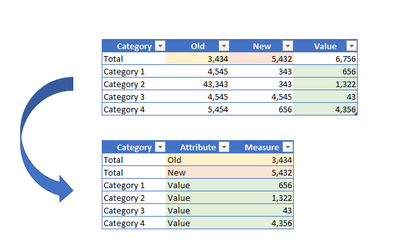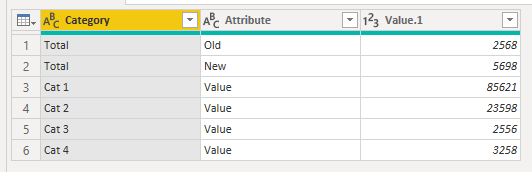cancel
Showing results for
Did you mean:Regular Visitor

## Unpivot selected columns based on certain conditions

Hi there,

I want to unpivot 3 value columns based on the row content given in another column.

Logic: If Category = "Total" unpivot columns Old and New Else unpivot column ValueHow to adjust this formula accordingly?Thank you very much!

BR
Nata

1 ACCEPTED SOLUTIONSuper User

Hi Nata,

Do the unpivot as normal, then just add a custom filter.

Paste this into a new blank query to see the steps to take. It's the conditions in the 'filterRows' step that do the work:

``````let
Source = Table.FromRows(Json.Document(Binary.Decompress(Binary.FromText("PY0xDsAgCEWv0jC7CIIw9wrdjIN7ky7eP/WbtNPP58GjNbqeOW5KxGq+Qi0QYuqFemp0jnlkAA8DwDyRq3H+OeMeJytzFNtVl+hbEAAuFYJaYr9T+zGM4SrwSCijCy9d7y8=", BinaryEncoding.Base64), Compression.Deflate)), let _t = ((type nullable text) meta [Serialized.Text = true]) in type table [Category = _t, Old = _t, New = _t, Value = _t]),
chgTypes = Table.TransformColumnTypes(Source,{{"Category", type text}, {"Old", Int64.Type}, {"New", Int64.Type}, {"Value", Int64.Type}}),

unpivOthCols = Table.UnpivotOtherColumns(chgTypes, {"Category"}, "Attribute", "Value.1"),

filterRows = Table.SelectRows(unpivOthCols, each  if [Category] = "Total" then [Attribute] <> "Value" else [Attribute] = "Value")

in
filterRows``````

Example query turns this:...into this:Pete

Now accepting Kudos! If my post helped you, why not give it a thumbs-up?

Proud to be a Datanaut!

2 REPLIES 2Regular Visitor

thank you so much Pete, works perfectly!Super User

Hi Nata,

Do the unpivot as normal, then just add a custom filter.

Paste this into a new blank query to see the steps to take. It's the conditions in the 'filterRows' step that do the work:

``````let
Source = Table.FromRows(Json.Document(Binary.Decompress(Binary.FromText("PY0xDsAgCEWv0jC7CIIw9wrdjIN7ky7eP/WbtNPP58GjNbqeOW5KxGq+Qi0QYuqFemp0jnlkAA8DwDyRq3H+OeMeJytzFNtVl+hbEAAuFYJaYr9T+zGM4SrwSCijCy9d7y8=", BinaryEncoding.Base64), Compression.Deflate)), let _t = ((type nullable text) meta [Serialized.Text = true]) in type table [Category = _t, Old = _t, New = _t, Value = _t]),
chgTypes = Table.TransformColumnTypes(Source,{{"Category", type text}, {"Old", Int64.Type}, {"New", Int64.Type}, {"Value", Int64.Type}}),

unpivOthCols = Table.UnpivotOtherColumns(chgTypes, {"Category"}, "Attribute", "Value.1"),

filterRows = Table.SelectRows(unpivOthCols, each  if [Category] = "Total" then [Attribute] <> "Value" else [Attribute] = "Value")

in
filterRows``````

Example query turns this:...into this:Pete

Now accepting Kudos! If my post helped you, why not give it a thumbs-up?

Proud to be a Datanaut!